## Thursday, October 13, 2016

### Math Family Facts – Multiply and Divide by 12

These slides feature multiplying and dividing by 12 by the use of math family facts, demonstrating the commutative property for multiplication. This is part of a series in which numbers one through twelve are multiplied and divided by one through twelve. Each number is multiplied by zero but not divided by zero.

These serve as a good review of information in which readers can look at patterns. The numbers are highlighted in different colors to make it easier to see how they are applied in the math equations.

Directions
1. Click on the first slide to enlarge.
2. Click on the slide or thumbnail to advance to the next slide.
Find more than 300 free resources at my Student Survive 2 Thrive blog. Thanks for visiting, and feel free to post comments in the comment section below the last slide.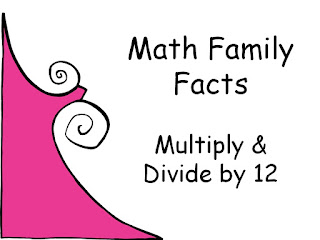Math Family Facts - Multiply and Divide by 12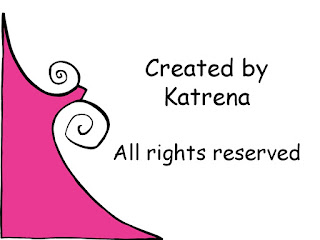Free Math Resources by KatrenaMath Family Facts for 12 and 0 and 012 X 0 = 0, 0 X 12 = 0Math Family Facts for 12 and 1 and 1212 X 1 = 12, 1 X 12 = 12, 12 Divided by 12 = 1, 12 Divided by 1 = 12Math Family Facts for 12 and 2 and 24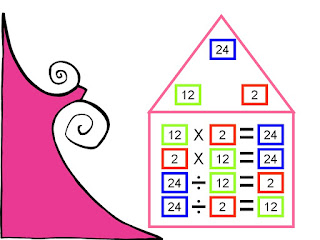12 X 2 = 24, 2 X 12 = 24, 24 Divided by 12 = 2, 24 Divided by 2 = 12Math Family Facts for 12 and 3 and 36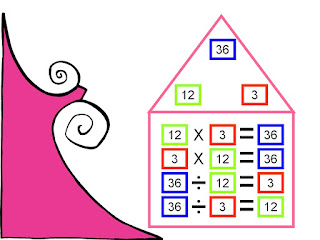12 X 3 = 36, 3 X 12 = 36, 36 Divided by 12 = 3, 36 Divided by 3 = 12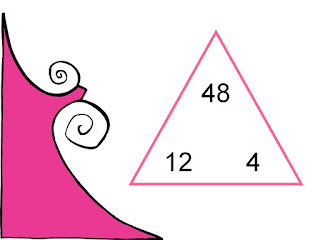Math Family Facts for 12 and 4 and 4812 X 4 = 48, 4 X 12 = 48, 48 Divided by 12 = 4, 48 Divided by 4 = 12Math Family Facts for 12 and 5 and 60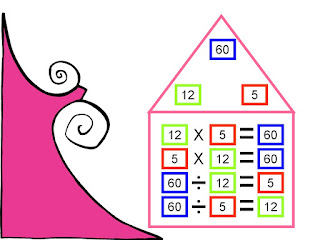12 X 5 = 60, 5 X 12 = 60, 60 Divided by 12 = 6, 60 Divided by 5 = 12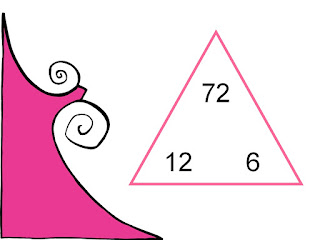Math Family Facts for 12 and 6 and 72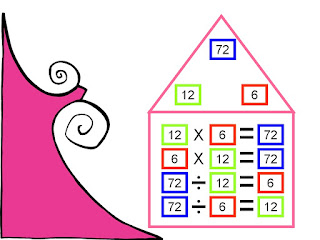12 X 6 = 72, 6 X 12 = 72, 72 Divided by 12 = 6, 72 Divided by 6 = 12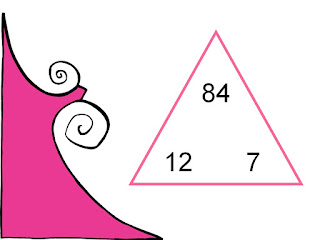Math Family Facts for 12 and 7 and 8412 X 7 = 84, 7 X 12 = 84, 84 Divided by 12 = 7, 84 Divided by 7 = 12Math Family Facts for 12 and 8 and 96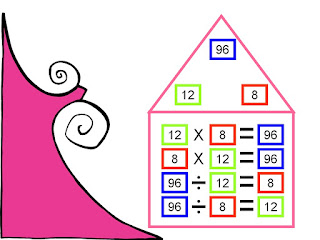12 X 8 = 96, 8 X 12 = 96, 96 Divided by 12 = 8, 96 Divided by 8 = 12Math Family Facts for 12 and 9 and 10812 X 9 = 108, 9 X 12 = 108, 108 Divided by 12 = 9, 108 Divided by 9 = 12Math Family Facts for 12 and 10 and 12012 X 10 = 120, 10 X 12 = 120, 120 Divided by 12 = 10, 120 Divided by 10 = 12Math Family Facts for 12 and 11 and 13212 X 11 = 132, 11 X 12 = 132, 132 Divided by 12 = 11, 132 Divided by 11 = 12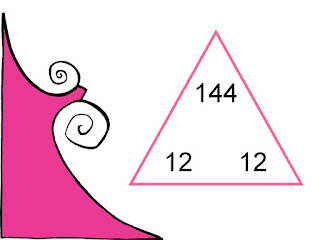Math Family Facts for 12 and 12 and 144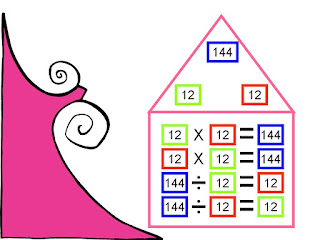12 X 12 = 144, 144 Divided by 12 = 12Free Math Resources at Student Survive 2 Thrive